Before I started writing this article, my knowledge of languages was summarized in: "I have no idea how this stuff works". And whenever I searched for a basic notion I felt I was reading Greek, I thought everything was magical, mystical. So if you don't understand anything about it either, I know exactly how you feel.

So I had the idea to learn about the topic to create this post. We are learning together. To try to demystify the topic, we will approach everything in practice. Let's start from zero and, at the end of this article, we will have a functional interpreter, running mathematical expressions, written in javascript.

I do not intend to go into too much depth, not least because this is a concept that has existed for several years (literally since the beginning of programming), and there are hundreds of thousands of technical terms, variations, algorithms and approaches. And also because I have basic knowledge on the topic. However, the goal is to move from "I have no idea how it works" to "aaah, so this is how it works". Do not expect that you will become a compiler ninja.

#### .css-1g84dnl{position:absolute;left:-26px;-webkit-text-decoration:none;text-decoration:none;color:rgba(255,255,255,0.05);-webkit-transition:color 300ms ease;transition:color 300ms ease;-webkit-user-select:none;-moz-user-select:none;-ms-user-select:none;user-select:none;}#Interpreter vs. Compiler

You may have noticed that I mixed the terms compiler and interpreter, and you may have got a knot in your head. And yes, this confusion usually happens. In fact, both are extremely similar, what differs is the end result. Both share the same concepts of construction of lexical, syntactic, semantic analysis, etc. The difference is that in the Interpreter, as the name suggests, the language will be interpreted in the end. That is, in our case, the javascript will decompose our input, translate according to the grammatical rules that we do, and even perform the calculations and return the result, all within the js. It will be a language running inside javascript, just like javascript runs inside C. Compilers, on the other hand, have a process of generating code, and it is more common in low-level languages, such as C, which compiles for Assembly.

## #Lexical Analysis

This is the first step of our interpreter, and is also known as "tokenizer", "scanner" or just "lexer". The purpose of this step is to extract the tokens from our entry. This is an important process for the "disambiguation" of language syntaxes. It is in this stage that they differ that == is one token (equality) and not two = tokens (attribution). In our case, we will not have any of these tokens mentioned because we will only work with mathematical expressions (+, -, *, /, (, ), and numbers).

#### #Token

Think of a token as a "useful" piece of our entry. It can be a number, a parenthesis, an operator. In more complete languages it can be a keyword, a variable, among others. At the beginning we only have a sequence of characters, which apparently do not mean anything, but at the end that piece of string that had 1 and then 0 was actually a token number of value 10. In our interpreter we will discard all empty spaces. All other characters will be part of the token, as in the example below: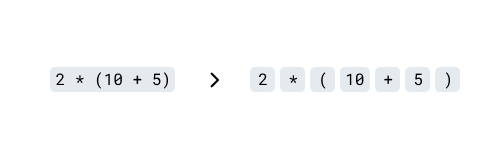Lexer's job is just the separation of tokens, it doesn't care if the syntax is broken (for example, if you have a parenthesis without closing, it won't care). Lexical errors are usually an unknown character. If I run 1 % 1, it will have no knowledge of what this % character is, and then, lexer should throw a lexical error.

We will represent a token as an object that has type and value properties (for literals), so we will have a list of tokens that looks like this:

``````[
{ type: "Number", value: 2 },
{ type: "Star" },
{ type: "LeftParen" },
{ type: "Number", value: 10 },
{ type: "Plus" },
{ type: "Number", value: 5 },
{ type: "RightParen" },
{ type: "End" }
];``````

#### #The lexer

The algorithm starts with the lexer going through the entire input string, char by char, and understanding when to save the token or continue the analysis.

``````const lexer = (input /*: string */) => {
let tokens = []; // where our tokens will be stored
let pointer = 0; // set the pointer to index 0, that is, at the beginning

while (pointer < input.length) {
// continues execution until the pointer is at the end of the input
let char = input[pointer]; // takes the char corresponding to the pointer pos

if (/* char is token A */) {
// performs logic based on the char found and
// advances the pointer to continue the execution of the while
tokens.push({ token: "A" });
pointer++;
}

if (/* char is B */) {
tokens.push({ token: "B" });
pointer++;
}

// at the end of the while
// throw an error for unhandled characters
throw new Error(`Unexpected char \${char}`);
}
};``````

See an example of a lexer running step-by-step by clicking in next:
(tip: you can edit the expression in the top input)

`(`
`5`
` `
`+`
` `
`2`
`)`
` `
`*`
` `
`1`
`0`
`↑`
`0`

### Instructions

To better understand the pointer, I explain: the string behaves very similar to an array in terms of indexes and iteration. We were able to access a character from the string by passing its position. That way, each time the pointer is incremented, we can read the next character in the string and apply the logic we want.

``````const input = "2 * (10 + 5)";
let pointer = 0;

input[pointer]; // input -> '2'

pointer++;
input[pointer]; // input -> ' '

pointer++;
input[pointer]; // input -> '*'``````

#### #Ignoring whitespaces

Whitespaces are essential for writing readable code, and in many cases they also have a syntactic role. In our case, they will just be ignored. So when our lexer finds a whitespace, it just goes on:

``````const lexer = input => {
let tokens = [];
let pointer = 0;

while (pointer < input.length) {
let char = input[pointer];

if (char === " ") {
// increase the pointer so that in the next execution
// of the while it takes the next char
// and stop the current execution of the while
pointer++;
continue;
}

throw new Error(`Unexpected char \${char}`);
}
};``````

#### #Dealing with single character tokens

Most of the tokens that we will use are of only one character (`*`, `+`, `(`, `)`, etc), so they are the simplest to parse, since it is not necessary to look at the front characters, only the current one within the iteration:

``````const lexer = input => {
let tokens = [];
let pointer = 0;

while (pointer < input.length) {
let char = input[pointer];

if (char === " ") {
pointer++;
continue;
}

if (char === "+") {
// adds the "Plus" token to our token list
tokens.push({ type: "Plus" });
pointer++;
continue;
}

throw new Error(`Unexpected char \${char}`);
}
};``````

After repeating the process with all the other tokens, we will have something like:

``````const lexer = input => {
let tokens = [];
let pointer = 0;

while (pointer < input.length) {
let char = input[pointer];

if (char === " ") {
pointer++;
continue;
}

if (char === "+") {
tokens.push({ type: "Plus" });
pointer++;
continue;
}

if (char === "-") {
tokens.push({ type: "Minus" });
pointer++;
continue;
}

if (char === "*") {
tokens.push({ type: "Star" });
pointer++;
continue;
}

if (char === "/") {
tokens.push({ type: "Slash" });
pointer++;
continue;
}

if (char === "(") {
tokens.push({ type: "LeftParen" });
pointer++;
continue;
}

if (char === ")") {
tokens.push({ type: "RightParen" });
pointer++;
continue;
}

throw new Error(`Unexpected char \${char}`);
}
};``````

#### #Dealing with multi-character tokens

There are tokens formed by more than one character, and in most languages this group forms most of the tokens, however, in our case it will only be the token Number.

`10 + 1000` `10` `+` `1000`

To cover this scenario, as soon as we find the first character of number, we will observe its next characters and go grouping as long as they remain numbers, and when we find any character that is not what we are looking for, we cancel the process and save the token with the final grouping.

``````const lexer = input => {
let tokens = [];
let pointer = 0;

while (pointer < input.length) {
let char = input[pointer];

// [...]

// let's use a simple regex to test if the char is a digit
if (/[0-9]/.test(char)) {
let value = "";

// while the char is a number, add no value and advance the pointer
while (/[0-9]/.test(char)) {
value += char;
pointer++;
char = input[pointer];
}

// when we break the previous while, we add the final result to the tokens
tokens.push({ type: "Number", value: parseInt(value) });

// this time we will not increment the pointer before continue because it
// is already at a point after the end number for breaking the grouping while
continue;
}

throw new Error(`Unexpected char \${char}`);
}
};``````

At the end of it, we add a token to indicate the end of our expression and return all tokens. We have our lexer:

``````const lexer = input => {
let tokens = [];
let pointer = 0;

while (pointer < input.length) {
let char = input[pointer];

if (char === " ") {
pointer++;
continue;
}

if (char === "+") {
tokens.push({ type: "Plus" });
pointer++;
continue;
}

if (char === "-") {
tokens.push({ type: "Minus" });
pointer++;
continue;
}

if (char === "*") {
tokens.push({ type: "Star" });
pointer++;
continue;
}

if (char === "/") {
tokens.push({ type: "Slash" });
pointer++;
continue;
}

if (char === "(") {
tokens.push({ type: "LeftParen" });
pointer++;
continue;
}

if (char === ")") {
tokens.push({ type: "RightParen" });
pointer++;
continue;
}

if (/[0-9]/.test(char)) {
let value = "";

while (/[0-9]/.test(char)) {
value += char;
pointer++;
char = input[pointer];
}

tokens.push({ type: "Number", value: parseInt(value, 10) });
continue;
}

throw new Error(`Unexpected char \${char}`);
}

tokens.push({ type: "End" });
};``````

## #Syntax Analisys

This step is also known as parsing, and it is the second phase of our interpreter. This is where we will analyze the tokens produced in the lexer to build a tree. Each interpreter has its objectives in this phase, it will depend on each purpose, but in general, most interpreters use this phase to generate hierarchical trees that facilitate a later analysis, both human and by a machine (through patterns such as Visitor Pattern, which we will address it later). Our interpreter will use this phase to generate an abstract syntax tree, better known as AST.

A particularity of the expressions that makes it more complex to solve is the precedence of the operators. It is already intuitive for us, but we learned it at school. Observe the expression below:

``4 + 3 * 2``

Your already knows that you need to solve multiplication first, then solve the sum. And you get the result easily. Our parser will also need to know this, and the best way to represent precedence is using trees: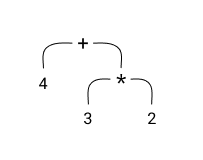When disassembling our expression, and assembling our tree, we start with the operators with the lowest precedence (in this case, the `+`), so that the operators with the highest prevalence (`*`) are at the end. For it is from the bottom up that we will solve our tree: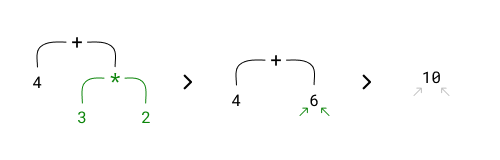### #Recursive Descent Parser

Okay, it looks simple, right? This is because the example is simple, but what if we mix unary operators (like negative)? Parentheses (subexpressions)? How to transform tokens into a tree considering different orders of precedence? Among several techniques and algorithms to deal with this parsing problem, there is the recursive descent, which is one of the best known for being both simple (at least simpler than others) and efficient. And that’s what I’m going to use to build our parser.

It is called recursive descent because it consists of several recursive functions that go in a descending order. These functions represent rules of a grammar, so for each rule of our grammar there is a function dedicated to solving it within our parser.

#### #Grammar

The concept of grammars is one of the pillars at this stage, and quite powerful. This is because grammars are able to define operations from tokens and at the same time define an order of priority (along with recursion), solving our precedence problem.

To better understand, imagine that a grammar is a set of rules within our expression. For example, multiplication is a rule within our grammar represented by the tokens of `Number`, `Star` and `Number`, in that sequence. Without this, there is no multiplication because I cannot multiply anything without having two values (two `Number`s), and in this case the `Star` to indicate that I need to do the multiplication.

Following the same logic, our addition rule would be the joining of the `Number`, `Plus` and `Number` tokens, right? Well, the idea is there, but not quite. Within the recursive parser we define the grammar in the reverse order of precedence – addition comes before the multiplication here, for the same reason that we started breaking the tree by the `+` operator at the beginning of this topic – only when we define a low precedence rule, we use the next rule (of higher precedence) as the definition value, as in the example below:

``````Addition = Multiplication "Plus" Multiplication
Multiplication = Number "Star" Number
Number = "Number"
``````

However, our expression may not have a multiplication, so how will it solve the addition? Let's adapt our multiplication rule to return an "or".

``Multiplication = (Number "Star" Number) OR Number``

That way, if there is no sequence of `number`, `Star` and `number`, it will only return the `number`, because we don't have a multiplication. We will do the same in addition, because we may not have an addition in our expression. Remember, a simple number (`5`) is already a valid expression.

``````Addition = (Multiplication "Plus" Multiplication) OR Multiplication
Multiplication = (Number "Star" Number) OR Number
Number = "Number"
``````

This definition means that when we create the algorithm, when looking for an addition, it will look for multiplications within the recursion. Remember that each of these rules will become functions in our parser, so for the example above we would have something like `add()` and `mul()`.

The addition function would look for multiplications to be solved before (since within a recursive function, the first executions use the value of the last executions, causing the multiplication to be solved before the addition).

A little confused, right? But let's get there. There is a notation for defining grammars, and it will help to facilitate understanding. They somewhat resemble regular expressions.

Here is the same example used above, in grammar notation: (I will explain the details below)

``````expr → add
mul  → num (STAR num)*
num  → NUMBER
``````

The rules when used in the definitions are called `nonterminals`, as they are not terminal, they have to be executed within the recursion until they become the terminals that are final values (our tokens are terminals). In `mul (PLUS mul)*`, `mul`s are the nonterminals, while `PLUS` is a terminal.

In our grammar, nonterminals are represented in lower case (`expr`, `add`, `mul`, `num`), while the terminals are represented in upper case (`PLUS`, `STAR`, `NUMBER`).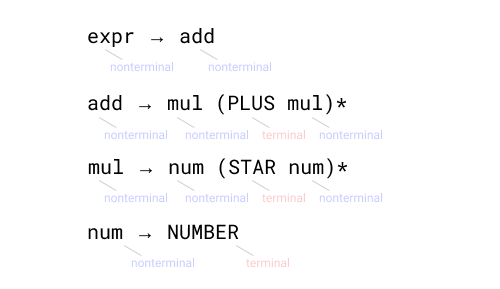As in regular expressions, parentheses are used for grouping, and the asterisk is used to say that that value (or grouping, in our case) can be repeated 0 or more times.

In other words, in the rule `mul → num (STAR num)*` I am saying that the `mul` will have a product that has `num` and may have an infinite repetition of `PLUS num`. Like this:

``````5           // num
5 * 5       // num (STAR num)
5 * 5 * 5   // num (STAR num) (STAR num)``````

As you can see, our `(STAR num)` representation are repeated zero or more times

#### #The parser

I know very well that just from theory, things are difficult to understand. I suffered for it. So let's start coding our parser based on the assumption that we only have addition and multiplication, for simplicity, and then we'll add the other operators.

``````const parser = tokens => {
let pointer = 0;

// ...
};``````

We started with the same premise as Lexer. We will use a pointer to cycle through tokens, but unlike lexer, we will create some support functions so that our parser is not so confused:

``````const parser = tokens => {
let pointer = 0;

const peek = () => tokens[pointer];
const prev = () => tokens[pointer - 1];
const match = type => {
if (peek().type === type) {
pointer++;
return true;
}
return false;
};
};``````

In parts: `peek` and `prev` functions are simple, they bring us the current token and the previous token, respectively, according to our pointer.

The `match` function will help us to identify the terminals by types from our pointer. So if you need to know if the pointer is in a Number type token, just use `match('Number')`, if the answer is yes, it will return true and advance the pointer, if not, it will just return false.

From here we can already build our recursive functions from the rules of our grammar. For now we are not going to set up an AST, but just solve the expression and get the final result.

Our `expr` rule is really as simple as the notation demonstrates: it returns the execution of `add` function that does not exist yet.

``````const parser = tokens => {
let pointer = 0;

const peek = () => tokens[pointer];
const prev = () => tokens[pointer - 1];
const match = type => {
if (peek().type === type) {
pointer++;
return true;
}
return false;
};

const expr = () => {
};
};``````

The `add` function rule will return a result that starts with `mul`, and as long as it finds the `PLUS` token, it will add to the next `mul`. The while here represents the asterisk of the notation very well.

``````const parser = tokens => {
let pointer = 0;

const peek = () => tokens[pointer];
const prev = () => tokens[pointer - 1];
const match = type => {
if (peek().type === type) {
pointer++;
return true;
}
return false;
};

const expr = () => {
};

// add → mul (PLUS mul)*
const add = () => {
// mul
let result = mul();

// (PLUS mul)*
while (match("Plus")) {
// if it finds the "Plus" token then it will add to the following `mul()`
result = result + mul();
}

return result;
};
};``````

The `mul` rule is identical to the add, but now it will use the num with the `STAR` operator.

``````const parser = tokens => {
let pointer = 0;

const peek = () => tokens[pointer];
const prev = () => tokens[pointer - 1];
const match = type => {
if (peek().type === type) {
pointer++;
return true;
}
return false;
};

const expr = () => {
};

const add = () => {
let result = mul();

while (match("Plus")) {
result = result + mul();
}

return result;
};

// mul → num (STAR num)*
const mul = () => {
// num
let result = num();

// (STAR num)*
while (match("Star")) {
result = result * num();
}

return result;
};
};``````

Finally our last rule. Since `NUMBER` is a terminal we have to ensure that it is the `Number` token, and return its token value, terminal in fact. And as it is the last rule of our parser, if it arrived here and it is not a `Number`, then we have a syntax error because it was not captured by any of our grammatical rules (for example 5 + * 3). For this we will throw a `Error`.

``````const parser = tokens => {
let pointer = 0;

const peek = () => tokens[pointer];
const prev = () => tokens[pointer - 1];
const match = type => {
if (peek().type === type) {
pointer++;
return true;
}
return false;
};

const expr = () => {
};

const add = () => {
let result = mul();

while (match("Plus")) {
result = result + mul();
}

return result;
};

const mul = () => {
let result = num();

while (match("Star")) {
result = result * num();
}

return result;
};

// num → NUMBER
const num = () => {
// NUMBER
if (match("Number")) {
return prev().value;
}

throw Error(`Syntax Error: Unexpected \${peek().type} token`);
};
};``````

In the end, the parser returns the execution of the first rule...

``return expr();``

...and the recursion does everything else:

``````const parser = tokens => {
let pointer = 0;

const peek = () => tokens[pointer];
const prev = () => tokens[pointer - 1];
const match = type => {
if (peek().type === type) {
pointer++;
return true;
}
return false;
};

const expr = () => {
};

// add  → mul (PLUS mul)*
const add = () => {
let result = mul();

while (match("Plus")) {
result = result + mul();
}

return result;
};

// mul  → num (STAR num)*
const mul = () => {
let result = num();

while (match("Star")) {
result = result * num();
}

return result;
};

// num  → NUMBER
const num = () => {
if (match("Number")) {
return prev().value;
}

throw new Error(`Syntax Error: Unexpected \${peek().type} token`);
};

return expr();
};``````

At this point you already have a parser running with precedence between `+` and `*`!

You can check the current parser we built in this link

#### #Subtraction and Division

As I said before, we only use addition and multiplication to simplify, but the time has come to add new operators. Here we will enter a new precedence rule for us. Unlike addition and multiplication, which have precedence among themselves, addition and subtraction do not. They are executed in the order they come. Just like multiplication and division. Therefore, we cannot separate both into different rules, because different rules represent different precedence.

Then we will use a simple "or" inside our token, so that it matches any of the two:

``````  expr → add
- add  → mul (PLUS mul)*
+ add  → mul ((PLUS | MINUS) mul)*
- mul  → num (STAR num)*
+ mul  → num ((STAR | SLASH) num)*
num  → NUMBER``````

Okay, now that we've updated our grammar, let's update our parser. As we only change the add and mul rules, it is only in these functions that we will change:

``````// add → mul ((PLUS | MINUS) mul)*

const add = () => {
// mul
let result = mul();

// ((PLUS | MINUS) mul)*
while (match("Plus") || match("Minus")) {
// we need to check if the token that matched is Plus or Minus to do the correct operation
// we use prev because the pointer has already advanced through the match
if (prev().type === "Plus") result = result + mul();
if (prev().type === "Minus") result = result - mul();
}

return result;
};``````
``````// mul → num ((STAR | SLASH) num)*

const mul = () => {
// num
let result = num();

// ((STAR | SLASH) num)*
while (match("Star") || match("Slash")) {
if (prev().type === "Star") result = result * num();
if (prev().type === "Slash") result = result / num();
}

return result;
};``````

You can check the current parser we built in this link

#### #Unary Operators

So far we only deal with binary operators, that is, when the operator needs two values to generate a result. However, there are operators that are unary, such as the negative number (-5) and the positive number (+5). Although the tokens are the same, they cannot be confused with subtraction and addition. Unary operations have a higher precedence than binary ones.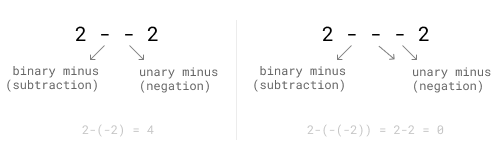Knowing this, we will add the unaries rule `(PLUS | MINUS)` number to our grammar.

``````  expr → add
add  → mul ((PLUS | MINUS) mul)*
- mul  → num ((STAR | SLASH) num)*
+ mul  → una ((STAR | SLASH) una)*
+ una  → ((PLUS | MINUS) una) | num
num  → NUMBER``````

First we need to change the `mul` rule, because the next rule is no longer `num`, but our new `una` rule. There is a peculiarity in this new rule, which is the fact that the unary operator can operate on other unary operators. Note: `- - 5`, this is a valid expression. They consist of two nested negative numbers, which results in a positive number 5 (minus minus equals plus). Just as the expression `- - + -5` is also valid. For this reason, in the one rule we use it itself as a nontermial after the operator.

So let's implement.

In the `mul` rule we will just replace the `num` by `una`:

``````// mul → una ((STAR | SLASH) una)*

const mul = () => {
// una
let result = una();

// ((STAR | SLASH) una)*
while (match("Star") || match("Slash")) {
if (prev().type === "Star") result = result * una();
if (prev().type === "Slash") result = result / una();
}

return result;
};``````

And our new `una` rule

``````// una → ((PLUS | MINUS) una) | num

const una = () => {
// ((PLUS | MINUS) una)
if (match("Plus") || match("Minus")) {
if (prev().type === "Plus") return +una();
if (prev().type === "Minus") return -una();
}

// | num
return num();
};``````

You can check the current parser we built in this link

#### #Subexpressions

Subexpressions - the parentheses - allow us, more than grouping, to break the line of precedence of expressions. They have the highest precedence within expressions, that is, they are calculated before any other. So if on the one hand, in this expression `4 + 3 * 2` the multiplication is calculated first, in this other `(4 + 3) * 2` the subexpression `4 + 3` is calculated first, and consequently, the addition as well. Bringing two different results. However, within subexpression, the order of precedence is still respected, for example in `(4 + 3 * 2) * 2`, although the parenthesis is calculated first, it will start with 3 * 2. Knowing this, note that subexpressions, as the name suggests, they are nothing more than whole expressions that will be executed before. We already have the rule that deals with the expressions `expr`, we just need to identify the subexpressions by the parenthesis tokens and use the rule.

``````  expr → add
add  → mul ((PLUS | MINUS) mul)*
mul  → una ((STAR | SLASH) una)*
- una  → ((PLUS | MINUS) una) | num
+ una  → ((PLUS | MINUS) una) | prim
- num  → NUMBER
+ prim → NUMBER | LEFTPAREN expr RIGHTPAREN``````

The only thing we did was add `| LEFTPAREN expr RIGHTPAREN` in the rule of num. But we also had to change the name because it didn't make sense here anymore. We use `prim` from primary. The name here is quite arbitrary, it could be anything. To change the name of `num` we also had to touch the rule of `una`.

Fix in `una` function:

``````- // | num
- return num()
+ // | prim
+ return prim()``````

Older `num`, now `prim` function:

``````// prim → NUMBER | LEFTPAREN expr RIGHTPAREN

const prim = () => {
// NUMBER
if (match("Number")) {
return prev().value;
}

// | LEFTPAREN expr RIGHTPAREN
if (match("LeftParen")) {
const result = expr();

if (match("RightParen")) {
return result;
}

throw Error(`Syntax Error: Expecting closing paren`);
}

throw Error(`Syntax Error: Unexpected \${peek().type} token`);
};``````

The idea is quite simple, when finding a `LEFTPAREN`, we save a result with the result of `expr()` and only return it if it finds a `RIGHTPAREN` that closes the subexpression, otherwise we have a "unexpected end of input" error (unclosed paren)

You can check the current parser we built in this link

## #Abstract Syntax Tree (AST)

If you remember, at the beginning of this topic of Syntax Analysis, I said that we would build our AST. But where is it? I skipped that part. Instead, we used the parser to interpret the code directly, something like this: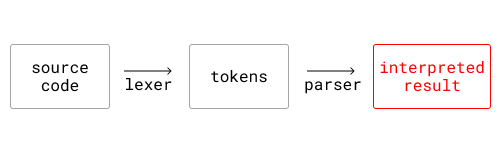I did this in order to better explain how the recursive descent parser works, without having to go into the details of AST. But the ideal is that we get to this: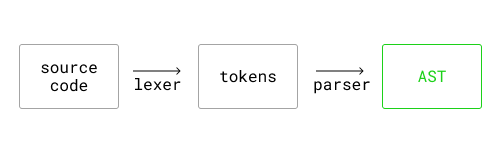But then you ask me: if we have already reached the final result, which was the result, why do we still need to go through AST? Well, in fact, if that was the only goal, we could simplify things and end here. But AST has a fundamental role in the third stage, the semantic analysis stage. And with AST we can not only calculate the final result of the expression, but do many other things.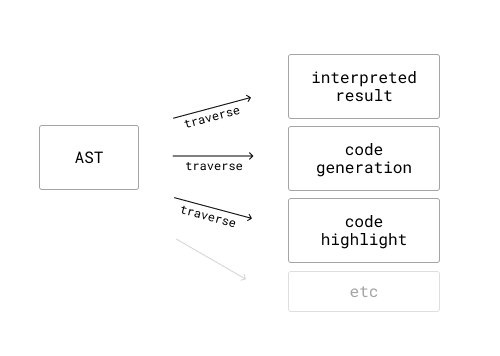But after all, what is AST? Okay, I know it's an abstract syntax tree, but, what does that mean? We know that a tree is a data structure, usually chained and represented by several nodes. Roughly speaking, we will take an expression and transform it into an object that represents this expression, as in this example:

From:

``1 + 2 * 3``

To:

``````{
"type": "BinaryOpNode",
"token": {
"type": "Plus"
},
"left": {
"type": "LiteralNode",
"token": {
"type": "Number",
"value": 1
}
},
"right": {
"type": "BinaryOpNode",
"token": {
"type": "Star"
},
"left": {
"type": "LiteralNode",
"token": {
"type": "Number",
"value": 2
}
},
"right": {
"type": "LiteralNode",
"token": {
"type": "Number",
"value": 3
}
}
}
}``````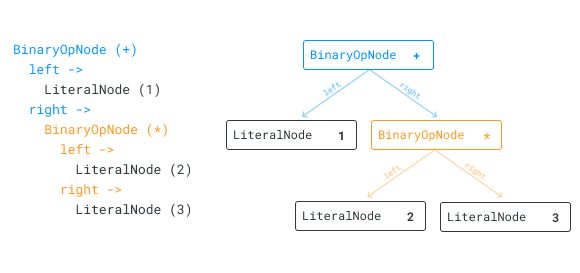#### #Nodes

Within the AST, each node represents a syntactic construction within the program. It can represent a literal, raw value, such as number, float, string, boolean, etc. It can represent an expression (with its factors and operator), a variable declaration (with the identifier and its assignment), among others. In our interpreter, we will have only three nodes, and they are:

The `LiteralNode` that will represent the literal nodes, in our case the number.

``````interface LiteralNode {
type: "LiteralNode";
token: Token;
}``````

The `BinaryOpNode` representing binary operators, that is, operators that have two factors (addition, subtraction, multiplication and division).

``````interface BinaryOpNode {
type: "BinaryOpNode";
left: Node;
right: Node;
token: Token;
}``````

The last node, `UnaryOpNode`, representing our unary operators, in our case the negative value.

``````interface UnaryOpNode {
type: "UnaryOpNode";
expr: Node;
token: Token;
}``````

Finally, we will create three functions that create our three nodes. They are simple functions that receive the data and return the object that represents the node.

``````const nodes = {
LiteralNode: ({ token }) =>
({ type: "LiteralNode", token }),

BinaryOpNode: ({ token, left, right }) =>
({ type: "BinaryOpNode", left, right, token }),

UnaryOpNode: ({ token, expr }) =>
({ type: "UnaryOpNode", expr, token })
};``````

#### #Back to the parser

Now that we have our nodes defined, we need to rewrite our parser so that it manages AST instead of interpreting the final result.

``````const add = () => {
let node = mul();

while (match("Plus") || match("Minus")) {
node = nodes.BinaryOpNode({ token: prev(), left: node, right: mul() });
}

return node;
};``````
``````const mul = () => {
let node = una();

while (match("Star") || match("Slash")) {
node = nodes.BinaryOpNode({ token: prev(), left: node, right: una() });
}

return node;
};``````
``````const una = () => {
if (match("Minus") || match("Plus")) {
return nodes.UnaryOpNode({ token: prev(), expr: una() });
}

return prim();
};``````
``````const prim = () => {
if (match("Number")) {
return nodes.LiteralNode({ token: prev() });
}

if (match("LeftParen")) {
const result = expr();

if (match("RightParen")) {
return result;
}

throw Error(`SyntaxError: Expecting closing paren`);
}

throw new Error(`SyntaxError: Unexpected \${peek().type} token`);
};``````

#### #Interpreting the AST

There are several ways that a language can do to obtain the final result of a source code. In general, it is often a complex part because it has to deal with contexts, scopes, globals, expressions, among others. Our interpreter only deals with expressions, so to execute our program we will just calculate our expression, and produce a final value.

Previously we had already reached the final value of our expression by calculating directly in the parser. We took a step back to rewrite it and produce an AST. Just like when we did it inside the parser, where we go through all the lexemes, identify nodes and calculate their value based on each type, we will now have to go through the AST and perform the same necessary logic for each node.

We will build a function that will visit each node, much like what a visitor pattern does, but much more simplistic and less flexible, for now.

``````const interpreter = ast => {
// defines the visitors of each node
const visitors = {
LiteralNode() {
/* ... */
},
BinaryOpNode() {
/* ... */
},
UnaryOpNode() {
/* ... */
}
};

// execute the visitor corresponding to the node
const visit = node => {
if (visitors[node.type]) {
return visitors[node.type](node, visit);
}
};

// execute the visitor of the first ast node
return visit(ast);
};``````

When we call our function `interpreter()`, it will go through all the nodes calling the visitor responsible for calculating its own result. For example, the visitor of `BinaryOpNode` will be responsible for adding the `left` and the `right` if his operator is of the `Plus` token. Or multiply the `left` and `right` if your operator is a `Star` token. The same idea for the other operators.

Each visitor receives the `node` as the first argument, and the visit function as the second argument, so that he can visit and get the result of his child nodes.

We start with `LiteralNode`, which is the simplest of nodes, since it represents a terminal node. It has no other nodes inside it, so to calculate its value we just return the value of your token.

``````const visitors = {
LiteralNode(node) {
return node.token.value;
}
};``````

We now create the visitor for `BinaryOpNode`. Although it seems complex, the idea is also simple. We will apply the logic to the `left` and `right` based on the operator. That is, for the sum operator, we will add the left and the right. But remember that `right` and `left` are also nodes, so we need to interpret them first.

``````const visitors = {
// ...
BinaryOpNode(node, visit) {
const leftVal = visit(node.left);
const rightVal = visit(node.right);

if (node.token.type === "Plus") {
return leftVal + rightVal;
}

if (node.token.type === "Minus") {
return leftVal - rightVal;
}

if (node.token.type === "Star") {
return leftVal * rightVal;
}

if (node.token.type === "Slash") {
return leftVal / rightVal;
}
}
};``````

For our last node, `UnaryOpNode`, the idea is not much different.

``````const visitors = {
// ...
UnaryOpNode(node, visit) {
const exprVal = visit(node.expr);

if (node.token.type === "Plus") {
return +exprVal;
}

if (node.token.type === "Minus") {
return -exprVal;
}
}
};``````

Done! We have an interpreter that uses AST as a base.
If we call `interpreter(parser(lexer("7 - 2 * 3"))` we get the value 1.

You can check the final parser we built in this link